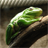# CalculatField code_block in VB.net. Please help

256
1
07-26-2012 05:34 AMby
New Contributor
I have trouble placing the VB script that works in the Calculate Field tool in the Toolbox in VB.net

Here is the VB script

dim getValue
if [FName] = "xxxx" then
getValue = [Distance] -40
else
getValue = [Distance]
end if

here is my VB.net code. I have trouble to place the "Code Block" in VB.net.

Dim gpCalField1 As ESRI.ArcGIS.DataManagementTools.CalculateField = New ESRI.ArcGIS.DataManagementTools.CalculateField()

Dim blockCode As String = "dim getValue" & vbNewLine & "If [FName] = " & """xxxx""" & "Then" & vbNewLine & "getValue =  [Distance] - 40" & vbNewLine    & "Else" & vbNewLine & "getValue = [Distance]" & vbNewLine & "End If"

gpCalField1.in_table = pgdbLocate & "\SurveyIntersect_Locate"
gpCalField1.field = "FSTA"
gpCalField1.expression = "getvalue"
'gpCalField1.code_block = """dim getValue If [FC_Name] = "" & ""Culvert"" & Then getValue = [MEAS] - 40  Else  getValue = [MEAS] End If"""
gpCalField1.code_block = blockCode
gpCalField1.expression_type = "VB"

gp.Execute(gpCalField1, Nothing)

Thank you in advance.

RH
Tags (5)
1 ReplyOccasional Contributor
You need a space before 'Then'.

Dim blockCode As String = "dim getValue" & vbNewLine & "If [FName] = " & """xxxx""" & " Then" & vbNewLine & "getValue = [Distance] - 40" & vbNewLine & "Else" & vbNewLine & "getValue = [Distance]" & vbNewLine & "End If"